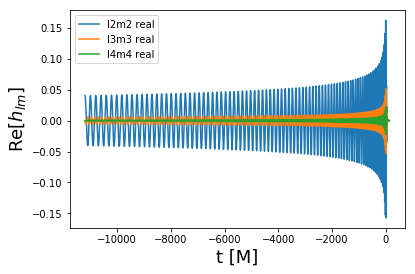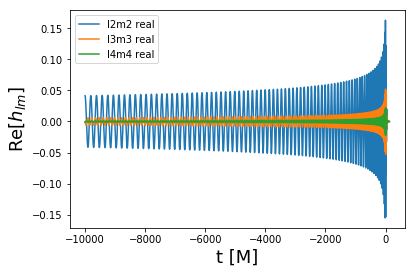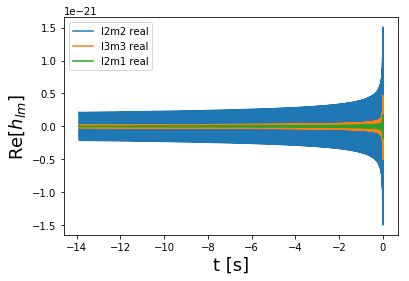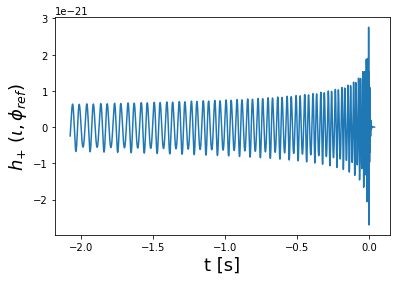# Example usage of NRHybSur3dq8 surrogate model.¶

In :
import numpy as np
import matplotlib.pyplot as P
%matplotlib inline

import gwsurrogate

setting __package__ to gwsurrogate.new so relative imports work
__name__ = gwsurrogate.new.spline_evaluation
__package__= gwsurrogate.new
setting __package__ to gwsurrogate.new so relative imports work
setting __package__ to gwsurrogate.new so relative imports work


## Download surrogate data, this only needs to be done once¶

In :
# This can take a few minutes
gwsurrogate.catalog.pull('NRHybSur3dq8')

Out:
'/Users/vijay/src/gwsurrogate/gwsurrogate/surrogate_downloadsNRHybSur3dq8.h5'

## Load the surrogate, this only needs to be done once at the start of a script¶

In :
sur = gwsurrogate.LoadSurrogate('NRHybSur3dq8')

Loaded NRHybSur3dq8 model


## Read the documentation¶

In :
help(sur)

Help on NRHybSur3dq8 in module gwsurrogate.surrogate object:

class NRHybSur3dq8(SurrogateEvaluator)
|  A class for the NRHybSur3dq8 surrogate model presented in Varma et al. 2018,
|  arxiv:1812.07865.
|
|  Evaluates gravitational waveforms generated by aligned-spin binary black hole
|  systems. This model was built using numerical relativity (NR) waveforms that
|  have been hybridized using post-Newtonian (PN) and effective one body (EOB)
|  waveforms.
|
|  This model includes the following spin-weighted spherical harmonic modes:
|  (2,2), (2,1), (2,0), (3,3), (3,2), (3,1), (3,0), (4,4) (4,3), (4,2) and (5,5).
|  The m<0 modes are deduced from the m>0 modes.
|
|  The parameter space of validity is:
|  q \in [1, 10] and chi1z/chi2z \in [-1, 1],
|  where q is the mass ratio and chi1z/chi2z are the spins of the heavier/lighter
|  BH, respectively, in the direction of orbital angular momentum.
|
|  The surrogate has been trained in the range
|  q \in [1, 8] and chi1z/chi2z \in [-0.8, 0.8], but produces reasonable waveforms
|  in the above range and has been tested against existing NR waveforms in that
|  range.
|
|  See the __call__ method on how to evaluate waveforms.
|  In the __call__ method, x must have format x = [q, chi1z, chi2z].
|
|  Method resolution order:
|      NRHybSur3dq8
|      SurrogateEvaluator
|      __builtin__.object
|
|  Methods defined here:
|
|  __init__(self, h5filename)
|
|  ----------------------------------------------------------------------
|  Methods inherited from SurrogateEvaluator:
|
|  __call__(self, q, chiA0, chiB0, M=None, dist_mpc=None, f_low=None, f_ref=None, dt=None, df=None, times=None, freqs=None, mode_list=None, ellMax=None, inclination=None, phi_ref=0, precessing_opts=None, tidal_opts=None, par_dict=None, units='dimensionless', skip_param_checks=False, taper_end_duration=None)
|      INPUT
|      =====
|      q :         Mass ratio, mA/mB >= 1.
|      chiA0:      Dimensionless spin vector of the heavier black hole, given in
|                  the coprecessing frame at reference epoch.
|      chiB0:      Dimensionless spin vector of the lighter black hole, given in
|                  the coprecessing frame at reference epoch.
|
|                  The coprecessing frame is the minimal rotation frame of
|                  arXiv:1110.2965, where the z-axis of this frame always tracks
|                  the direction of the instantaneous orbital angular momentum.
|                  For nonprecessing models, the direction of orbital angular
|                  momentum is constant and this is the same as the inertial
|                  frame.
|
|      M, dist_mpc: Either specify both M and dist_mpc or neither.
|          M        :  Total mass (solar masses). Default: None.
|          dist_mpc :  Distance to binary system (MegaParsecs). Default: None.
|
|      f_low :     Instantaneous initial frequency of the (2, 2) mode. In
|                  practice, this is estimated to be twice the initial orbital
|                  frequency in the coprecessing frame.
|                  Should be in cycles/M if units = 'dimensionless', should be in
|                  Hertz if units = 'mks'.
|                  If 0, the entire waveform is returned.
|                  Default: None, must be specified by user.
|                  WARNING: Using f_low=0 with a small dt (like 0.1M) can lead to
|                  very expensive evaluation for hybridized surrogates.
|
|      f_ref:      Frequency used to set the reference epoch at which the
|                  reference frame is defined and the spins are specified.
|                  See below for definition of the reference frame.
|                  Should be in cycles/M if units = 'dimensionless', should be
|                  in Hertz if units = 'mks'.
|                  Default: If f_ref is not given, we set f_ref = f_low. If
|                  f_low is 0, this corresponds to the initial index.
|
|                  For time domain models, f_ref is used to determine a t_ref,
|                  such that the orbital frequency in the coprecessing frame
|                  equals f_ref/2 at t=t_ref.
|
|      dt, df :    Time/Frequency step size, specify at most one of dt/df,
|                  depending on whether the surrogate is a time/frequency domain
|                  surrogate.
|                  Default: None. If None, the internal domain of the surrogate is
|                  used, which can be nonuniformly sampled.
|                  dt (df) Should be in M (cycles/M) if units = 'dimensionless',
|                  should be in seconds (Hertz) if units = 'mks'. Do not specify
|                  times/freqs if using dt/df.
|
|
|      times, freqs:
|                  Array of time/frequency samples at which to evaluate the
|                  waveform, depending on whether the surrogate is a
|                  time/frequency domain surrogate. time (freqs) should be in
|                  M (cycles/M) if units = 'dimensionless', should be in
|                  seconds (Hertz) if units = 'mks'. Do not specify dt/df if
|                  using times/freqs. Default None.
|
|      ellMax:     Maximum ell index for modes to include. All available m
|                  indicies for each ell will be included automatically.
|                  Default: None, in which case all available modes wll be
|                  included.
|
|      mode_list : A list of (ell, m) modes tuples to be included.
|                  Example: mode_list = [(2,2),(2,1)].
|                  Default: None, in which case all available modes are included.
|                  The m<0 modes will automatically be included for nonprecessing
|                  models.
|
|                  None: mode_list is allowed only for nonprecessing models; for
|                  precessing models use ellMax. For precessing systems, all m
|                  indices of a given ell index mix with each other, so there is
|                  no clear hierarchy. To get the individual modes just don't
|                  specify inclination and a dictionary of modes will be returned.
|
|      phi_ref :   Orbital phase at reference epoch. Default: 0.
|
|      inclination : Inclination angle between the orbital angular momentum
|                  direction at the reference epoch and the line-of-sight to the
|                  observer. If inclination is None, the mode data is returned as
|                  a dictionary. If specified, the complex strain (h = hplus -i
|                  hcross) evaluated at (inclination, pi/2) on the sky of the
|                  reference frame is returned. See below for definition of the
|                  reference frame. Default: None.
|
|      precessing_opts:
|                  A dictionary containing optional parameters for a precessing
|                  surrogate model. Default: None.
|                  Allowed keys are:
|                  init_quat: The initial unit quaternion (length 4 vector)
|                      giving the rotation from the coprecessing frame to the
|                      inertial frame at the reference epoch.
|                      Default: None, in which case the spins in the coprecessing
|                      frame are equal to the spins in the inertial frame.
|                  return_dynamics:
|                      Return the frame dynamics and spin evolution along with
|                      the waveform. Default: False.
|                  Example: precessing_opts = {
|                                      'init_quat': [1,0,0,0],
|                                      'return_dynamics': True
|                                      }
|
|      tidal_opts:
|                  A dictionary containing optional parameters for a tidal
|                  surrogate model. Default: None.
|                  Allowed keys are:
|                  Lambda1: The tidal deformability parameter for the heavier
|                      object.
|                  Lambda2: The tidal deformability parameter for the lighter
|                      object.
|                  Example: tidal_opts = {'Lambda1': 200, 'Lambda2': 300}
|
|
|      par_dict:   A dictionary containing any additional parameters needed for a
|                  particular surrogate model. Default: None.
|
|      units:      'dimensionless' or 'mks'. Default: 'dimensionless'.
|                  If 'dimensionless': Any of f_low, f_ref, dt, df, times and
|                      freqs, if specified, must be in dimensionless units. That
|                      is, dt/times should be in units of M, while f_ref, f_low
|                      and df/freqs should be in units of cycles/M.
|                      M and dist_mpc must be None. The waveform and domain are
|                      returned as dimensionless quantities as well.
|                  If 'mks': Any of f_low, f_ref, dt, df, times and freqs, if
|                      specified, must be in MKS units. That is, dt/times should
|                      be in seconds, while f_ref, f_low and df/freqs should be
|                      in Hz. M and dist_mpc must be specified. The waveform and
|                      domain are returned in MKS units as well.
|
|
|      skip_param_checks :
|                  Skip sanity checks for inputs. Use this if you want to
|                  extrapolate outside allowed range. Default: False.
|
|      taper_end_durataion:
|                  Taper the last TAPER_END_DURATION (M) of a time-domain waveform
|                  in units of M. For exmple, passing 40 will taper the last 40M.
|                  When set to None, no taper is applied
|                  Default: None.
|
|      RETURNS
|      =====
|
|      domain, h, dynamics
|
|
|      domain :    Array of time/frequency samples corresponding to h and
|                  dynamics, depending on whether the surrogate is a
|                  time/frequency domain model. This is the same as times/freqs
|                  if times/freqs are given as an inputs.
|                  For time domain models the time is set to 0 at the peak of
|                  the waveform. The time (frequency) values are in M (cycles/M)
|                  if units = 'dimensionless', they are in seconds (Hertz) if
|                  units = 'mks'
|
|      h :         The waveform.
|                      If inclination is specified, the complex strain (h = hplus
|                      -i hcross) evaluated at (inclination, pi/2) on the sky of
|                      the reference frame is returned. This follows the LAL
|                      convention, see below for details.  This includes all modes
|                      given in the ellMax/mode_list argument. For nonprecessing
|                      systems the m<0 modes are automatically deduced from the
|                      m>0 modes. To see if a model is precessing check
|                      self.keywords.
|
|                      Else, h is a dictionary of available modes with (l, m)
|                      tuples as keys. For example, h22 = h[(2,2)].
|
|                      If M and dist_mpc are given, the physical waveform
|                      at that distance is returned. Else, it is returned in
|                      code units: r*h/M extrapolated to future null-infinity.
|
|      dynamics:   A dict containing the frame dynamics and spin evolution. This
|                  is None for nonprecessing models. This is also None if
|                  return_dynamics in precessing_opts is False (Default).
|
|                  The dynamics include (L=len(domain)):
|
|                  q_copr = dynamics['q_copr']
|                      The quaternion representing the coprecessing frame with
|                      shape (4, L)
|                  orbphase = dynamics['orbphase']
|                      The orbital phase in the coprecessing frame with length L.
|                  chiA = dynamics['chiA']
|                      The time-dependent inertial frame chiA with shape (L, 3)
|                  chiB = dynamics['chiB']
|                      The time-dependent inertial frame chiB with shape (L, 3)
|
|
|      IMPORTANT NOTES:
|      ===============
|
|      The reference frame (or inertial frame) is defined as follows:
|          The +ve z-axis is along the orbital angular momentum at the reference
|          epoch. The orbital phase at the reference epoch is phi_ref. This means
|          that the separation vector from the lighter BH to the heavier BH is at
|          an azimuthal angle phi_ref from the +ve x-axis, in the orbital plane at
|          the reference epoch. The y-axis completes the right-handed triad. The
|          reference epoch is set using f_ref.
|
|          Now, if inclination is given, the waveform is evaluated at
|          (inclination, pi/2) in the reference frame. This agrees with the LAL
|          convention. See Harald Pfeiffer's, LIGO DCC document T18002260-v1 for
|          the LAL frame diagram.
|
|  ----------------------------------------------------------------------
|  Data descriptors inherited from SurrogateEvaluator:
|
|  __dict__
|      dictionary for instance variables (if defined)
|
|  __weakref__
|      list of weak references to the object (if defined)



## Evaluate the waveform¶

### Evaluate waveform modes in dimensionless units (default)¶

In :
q = 7
chiA = [0, 0, 0.5]
chiB = [0, 0, -0.7]
dt = 0.1        # step size, Units of M
f_low = 5e-3    # initial frequency, Units of cycles/M
t, h, dyn = sur(q, chiA, chiB, dt=dt, f_low=f_low)        # dyn stands for dynamics and is always None for this model

In :
# Let's see all available modes (m<0 modes will be included automatically if inclination/phi_ref arguments are given)
print sorted(h.keys())

[(2, 0), (2, 1), (2, 2), (3, 0), (3, 1), (3, 2), (3, 3), (4, 2), (4, 3), (4, 4), (5, 5)]

In :
P.plot(t, h[(2,2)].real, label='l2m2 real')
P.plot(t, h[(3,3)].real, label='l3m3 real')
P.plot(t, h[(4,4)].real, label='l4m4 real')
P.ylabel('Re[$h_{lm}$]', fontsize=18)
P.xlabel('t [M]', fontsize=18)
P.legend()

Out:
<matplotlib.legend.Legend at 0x11019a090>### Evaluate waveform on a fixed time array¶

In :
q = 7
chiA = [0, 0, 0.5]
chiB = [0, 0, -0.7]
f_low = 0  # this will be ignored and the wavefrom will be returned on the times given below
times = np.arange(-10000,130,0.1)
# The returned times are the same as the input times
times, h, dyn = sur(q, chiA, chiB, times=times, f_low=f_low)

P.plot(times, h[(2,2)].real, label='l2m2 real')
P.plot(times, h[(3,3)].real, label='l3m3 real')
P.plot(times, h[(4,4)].real, label='l4m4 real')
P.ylabel('Re[$h_{lm}$]', fontsize=18)
P.xlabel('t [M]', fontsize=18)
P.legend()

Out:
<matplotlib.legend.Legend at 0x1101bba90>### Evaluate waveform modes in physical units¶

In :
q = 7
chiA = [0, 0, 0.5]
chiB = [0, 0, -0.7]
M = 20             # Total masss in solar masses
dist_mpc = 100     # distance in megaparsecs
dt = 1./4096       # step size in seconds
f_low = 20         # initial frequency in Hz
t, h, dyn = sur(q, chiA, chiB, dt=dt, f_low=f_low, mode_list=[(2,2), (2,1), (3, 3)], M=M, dist_mpc=dist_mpc, units='mks')

P.plot(t, h[(2,2)].real, label='l2m2 real')
P.plot(t, h[(3,3)].real, label='l3m3 real')
P.plot(t, h[(2,1)].real, label='l2m1 real')
P.ylabel('Re[$h_{lm}$]', fontsize=18)
P.xlabel('t [s]', fontsize=18)
P.legend()

Out:
<matplotlib.legend.Legend at 0x1a31ca7990>### Evaluate waveform at a point on the sky¶

In :
q = 7
chiA = [0, 0, 0.5]
chiB = [0, 0, -0.7]
M = 60             # Total masss in solar masses
dist_mpc = 100     # distance in megaparsecs
dt = 1./4096       # step size in seconds
f_low = 20         # initial frequency in Hz
inclination = np.pi/4
phi_ref = np.pi/5

# Will only include modes given in mode_list argument as well as the m<0 counterparts.
# If mode_list is not specified, uses all available modes.
# Returns h_+ -i h_x
t, h, dyn = sur(q, chiA, chiB, dt=dt, f_low=f_low, mode_list=[(2,2), (2,1), (3, 3)], M=M, dist_mpc=dist_mpc,
inclination=inclination, phi_ref=phi_ref, units='mks')

P.plot(t, h.real)
P.ylabel('$h_{+}$ $(\iota, \phi_{ref})$', fontsize=18)
P.xlabel('t [s]', fontsize=18)

Out:
Text(0.5,0,'t [s]')In [ ]: